# Solution assignment 08 Unit circle and simple formulas

### Assignment 8

Show: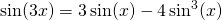### Solution

To show this relation we have to use a formula which is less often used (see Addition and Subtraction formulas):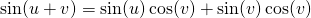Using this formula we can write the function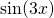as follows: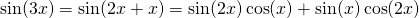In the summary formulas are given for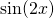andwhich can better be known by heart. We mean the following formulas: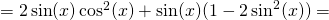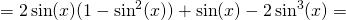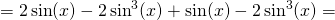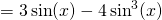0